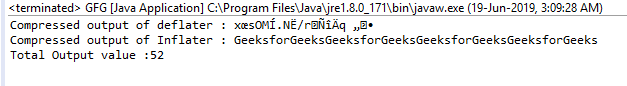Skip to content
Related Articles
Inflater getTotalOut() function in Java with examples
• Last Updated : 24 Jun, 2019

The getTotalOut() function of the Inflater class returns the total number of uncompressed bytes Output provided till now.

Function Signature:

```public int getTotalOut()
```

Syntax:

```i.getTotalOut();
```

Parameter: The function requires no parameter

Return Type: The function returns int value which is the total number of uncompressed bytes Output.

Exception: The function does not throw any exception

Example 1: use of getTotalOut() function

 `// Java program to describe the use``// of getTotalOut() function`` ` `import` `java.util.zip.*;``import` `java.io.UnsupportedEncodingException;`` ` `class` `GFG {``    ``public` `static` `void` `main(String args[])``        ``throws` `UnsupportedEncodingException,``               ``DataFormatException``    ``{`` ` `        ``// compress the data`` ` `        ``// deflater``        ``Deflater d = ``new` `Deflater();`` ` `        ``// get the text``        ``String pattern = ``"GeeksforGeeks"``, text = ``""``;`` ` `        ``// generate the text``        ``for` `(``int` `i = ``0``; i < ``4``; i++)``            ``text += pattern;`` ` `        ``// set the input for deflator``        ``d.setInput(text.getBytes(``"UTF-8"``));`` ` `        ``// finish``        ``d.finish();`` ` `        ``// output bytes``        ``byte` `output[] = ``new` `byte``[``1024``];`` ` `        ``// compress the data``        ``int` `size = d.deflate(output);`` ` `        ``// end``        ``d.end();`` ` `        ``// end of compression`` ` `        ``// use Inflater to get back the original data`` ` `        ``// Inflater``        ``Inflater i = ``new` `Inflater();`` ` `        ``// set the input for inflator``        ``i.setInput(output);`` ` `        ``// output bytes``        ``byte` `inflater_output[] = ``new` `byte``[``1024``];`` ` `        ``// uncompress the data``        ``int` `org_size = i.inflate(inflater_output);`` ` `        ``// output of inflater and deflater``        ``System.out.println(``"Compressed output of deflater : "``                           ``+ ``new` `String(output));``        ``System.out.println(``"Compressed output of Inflater : "``                           ``+ ``new` `String(inflater_output, ``"UTF-8"``));`` ` `        ``// get the total number of uncompressed bytes Output so far``        ``System.out.println(``"Total Output value :"` `+ i.getTotalOut());`` ` `        ``// end``        ``i.end();``    ``}``}`

Output:Note: The problem arises when the length of the Output is greater than Integer.MAX_VALUE then the result may overflow, we should use getBytesRead() function instead

Attention reader! Don’t stop learning now. Get hold of all the important Java Foundation and Collections concepts with the Fundamentals of Java and Java Collections Course at a student-friendly price and become industry ready. To complete your preparation from learning a language to DS Algo and many more,  please refer Complete Interview Preparation Course.

My Personal Notes arrow_drop_up